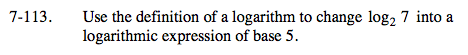Home > A2C > Chapter 7 > Lesson 7.2.2 > Problem7-113

7-113.

Use the definition of a logarithm to change log27 into a logarithmic expression of base 5. Homework Help ✎Start with the definition of the logarithm.
log27 = x
2x = 7

Take the log base 5 of both sides.
log5(2x) = log57

Use the power property.
x(log52) = log57

$\log_{2}7 =\frac{\log_{5}7}{\log_{5}2}$

Notice that this answer shows a very quick way to change bases.